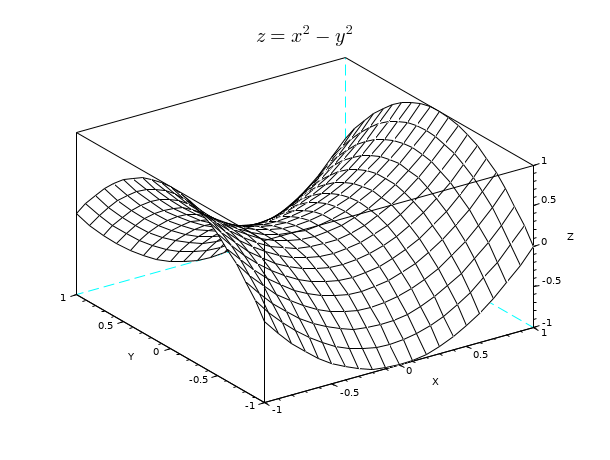Change language to:
Français - 日本語 - Português - Русский

See the recommended documentation of this function

Scilab Help >> Graphics > 3d_plot > mesh

# mesh

3D mesh plot

### Syntax

```mesh(Z)
mesh(X,Y,Z)
mesh(...,<GlobalProperty>)
mesh(...,<color>,<GlobalProperty>)
mesh(<axes_handle>,...)```

### Arguments

Z

a real matrix defining the surface height. It can not be omitted. The `Z` data is a `m`-by-`n` matrix.

X, Y

two real matrices : always set together, these data defines a new standard grid. This new `X` and `Y` components of the grid must match `Z` dimensions (see description below).

color

an optional real matrix defining a color value for each `(X(j),Y(i))` point of the grid (see description below).

<GlobalProperty>

this optional argument represents a sequence of couple statements `{PropertyName,PropertyValue}` that defines global objects' properties applied to all the curves created by this plot. For a complete view of the available properties (see GlobalProperty).

<axes_handle>

this optional argument forces the plot to appear inside the selected axes given by `axes_handle` rather than the current axes (see gca).

### Description

`mesh` draws a parametric surface using a rectangular grid defined by `X` and `Y` coordinates (if `{X,Y}` are not specified, this grid is determined using the dimensions of the `Z` matrix); at each point of this grid, a z coordinate is given using the `Z` matrix. `mesh` is based on the `surf` command with default option `color_mode` = white index (inside the current colormap) and `color_flag` = 0.

Data entry specification :

In this paragraph and to be more clear, we won't mention `GlobalProperty` optional arguments as they do not interfere with entry data (except for `"Xdata"`, `"Ydata"` and `"Zdata"` property, see GlobalProperty). It is assumed that all those optional arguments could be present too.

If `Z` is the only matrix specified, `mesh(Z)` plots the matrix `Z` versus the grid defined by `1:size(Z,2)` along the x axis and `1:size(Z,1)` along the y axis.

### Remarks

To enable the transparency mode you should set the `color_mode` option to 0.

### Examples

```[X,Y]=meshgrid(-1:.1:1,-1:.1:1);
Z=X.^2-Y.^2;
xtitle('\$\huge z=x^2-y^2\$');
mesh(X,Y,Z);```